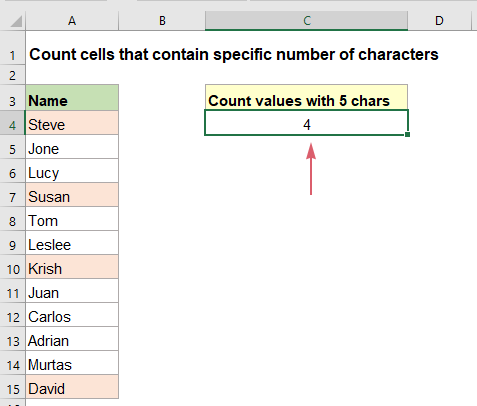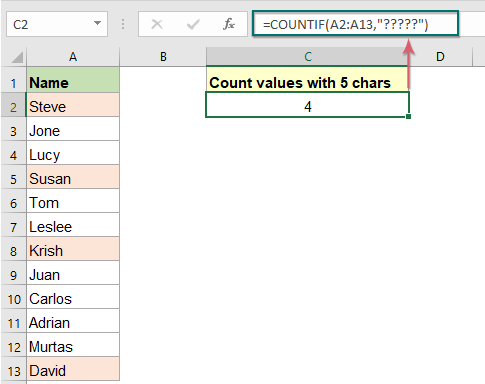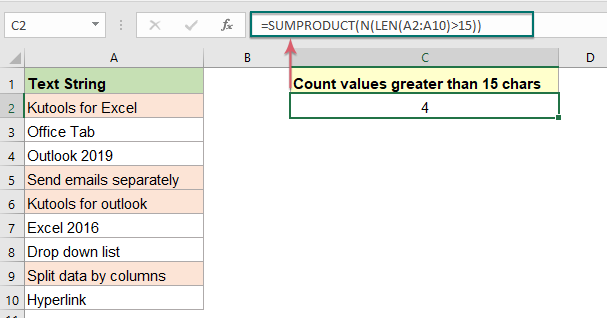## Count number of cells that contain specific number of characters

If you want to count the number of cells that contain a certain number of characters (such as 5 characters) in a range of cells as below screenshot shown. In Excel, the COUNTIF function can do you a favor. In this article, I will talk about how to get number of cells that contain exactly x characters or cells that contain more than a certain number of characters.#### Count number of cells that contain exactly x characters

The COUNTIF function can help you to get the number of cells that contains a specific number of characters, the generic syntax is:

=COUNTIF(range,"?????")
=COUNTIF(range,REPT(“?”,X))
• range: The range of cells that you want to count;
• ?: Indicates a wildcard matching any single character;
• X: The number of characters to count based on.

1. Please enter or copy any of the following formulas into a blank cell to output the result:

=COUNTIF(A2:A12,"?????")
=COUNTIF(A2:A13,REPT("?",5))

Note: In the first formula, ????? indicates any five characters that you want to count based on; In the second formula, REPT(“?”,5) will return a text string made of exactly 5 question marks. In this example REPT("?",5) returns "?????".

2. And then, press Enter key to get the result you need, see screenshot:#### Count number of cells with text length greater than x characters

If you want to get the number of cells that the text length is greater than 15 characters, you can apply the SUMPRODUCT, LEN, and N functions together, the generic syntax is:

=SUMPRODUCT(N(LEN(range)>X))
• range: The range of cells that you want to count;
• X: The number of characters to count based on.

1. Please enter or copy the below formula into a blank cell:

=SUMPRODUCT(N(LEN(A2:A10)>15))

2. Then press Enter key to get the result at once, see screenshot:##### Explanation of the formula:
• LEN(A2:A10): This LEN function calculates the length for each cell of selected range, and it returns the array list as this: {17;10;12;22;19;10;14;21;9}.
• LEN(A2:A10)>15: This part compares the text length of each cell with the specific number 15, if grater than 15, it displays TRUE, otherwise, it returns FALSE. It will get the result as this: {TRUE;FALSE;FALSE;TRUE;TRUE;FALSE;FALSE;TRUE;FALSE}.
• N(LEN(A2:A10)>15): This N function is used to convert TRUE and FALSE to 1 and 0 respectively, like this: {1;0;0;1;1;0;0;1;0}
• SUMPRODUCT(N(LEN(A2:A10)>15))=SUMPRODUCT({1;0;0;1;1;0;0;1;0}): At last, this SUMPRODUCT function will add up all numbers in the array, so you will get the result: 4.

#### Relative function used:

• COUNTIF:
• The COUNTIF function is a statistical function in Excel which is used to count the number of cells that meet a criterion.
• SUMPRODUCT:
• The SUMPRODUCT function can be used to multiply two or more columns or arrays together, and then get the sum of products.
• LEN:
• The LEN function returns the number of characters in a text string.
• N:
• The N function converts a value to a number.

#### More articles:

• Count number of cells not equal to many values in Excel
• In Excel, you may easily get the number of cells not equal to a specific value by using the COUNTIF function, but have you ever tried to count the cells which are not equal to many values? For example, I want to get the total number of the products in column A but exclude the specific items in C4:C6 as below screenshot shown. This article, I will introduce some formulas for solving this job in Excel.
• Count Number Of Cells That Contain Odd Or Even Numbers
• As we all known, odd numbers have a remainder of 1 when divided by 2, and the even numbers have a remainder of 0 when divided by 2. This tutorial, I will talk about how to get the number of cells that contain odd or even numbers in Excel.
• Count Number Of Cells Contain Numeric Or Non-Numeric Values
• If you have a range of data which contains both numeric and non- numeric values, and now, you may want to count the number of numeric or non-numeric cells as below screenshot shown. This article, I will talk about some formulas for solving this task in Excel.

### The Best Office Productivity Tools

#### Kutools for Excel - Helps You To Stand Out From Crowd

 Popular Features: Find, Highlight or Identify Duplicates  |  Delete Blank Rows  |  Combine Columns or Cells without Losing Data  |  Round without Formula ... Super VLookup: Multiple Criteria  |  Multiple Value  |  Across Multi-Sheets  |  Fuzzy Lookup... Adv. Drop-down List: Easy Drop Down List  |  Dependent Drop Down List  |  Multi-select Drop Down List... Column Manager: Add a Specific Number of Columns  |  Move Columns  |  Toggle Visibility Status of Hidden Columns  |  Compare Columns to Select Same & Different Cells ... Featured Features: Grid Focus  |  Design View  |  Big Formula Bar  |  Workbook & Sheet Manager | Resource Library (Auto Text)  |  Date Picker  |  Combine Worksheets  |  Encrypt/Decrypt Cells  |  Send Emails by List  |  Super Filter  |  Special Filter (filter bold/italic/strikethrough...) ... Top 15 Toolsets:  12 Text Tools (Add Text, Remove Characters ...)  |  50+ Chart Types (Gantt Chart ...)  |  40+ Practical Formulas (Calculate age based on birthday ...)  |  19 Insertion Tools (Insert QR Code, Insert Picture from Path ...)  |  12 Conversion Tools (Numbers to Words, Currency Conversion ...)  |  7 Merge & Split Tools (Advanced Combine Rows, Split Excel Cells ...)  |  ... and more

Kutools for Excel Boasts Over 300 Features, Ensuring That What You Need is Just A Click Away...#### Office Tab - Enable Tabbed Reading and Editing in Microsoft Office (include Excel)

• One second to switch between dozens of open documents!
• Reduce hundreds of mouse clicks for you every day, say goodbye to mouse hand.
• Increases your productivity by 50% when viewing and editing multiple documents.
• Brings Efficient Tabs to Office (include Excel), Just Like Chrome, Edge and Firefox.